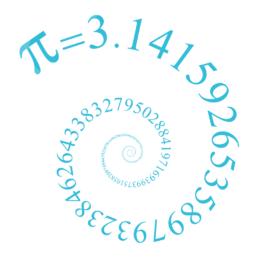Bournemouth Maths Tutor Barney M-THOW MANY SQUARES ARE THERE IN THIS GRID?

Before we see the quickest way to answer the question (scroll down if you are an expert!), let’s see some different possible approaches: GCSE APPROACH: There are 16 little squares.There is the one large square (the entire grid).But there are also some “2 by 2” squares, like the red one – I count 9 of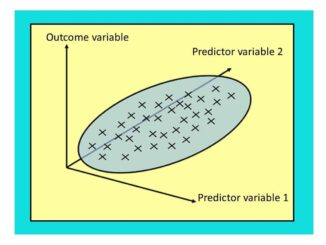Regression: lines (and planes) of best fit.

Let’s suppose we have some data for height and weight of some adult humans. We suspect the weight depends on the height – so taller people tend to weigh more than shorter. How can we develop this theory and use it to predict someone’s weight based on their height? Here’s a short introduction. AT GCSE:What does “MATH ERROR” mean?

SHORT ANSWER: this means you have just asked the calculator to do a sum it doesn’t like. The three most common reasons for getting this error message are: 1) you have tried to divide by zero: forbidden in maths! If you type in $3\div 0$ you are asking the calculator “zero times by what equals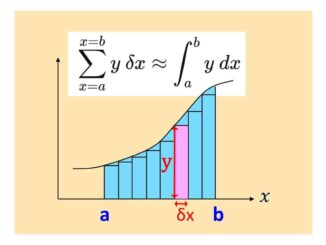WHAT’S ZERO TIMES BY INFINITY?

SHORT ANSWER: 0 x ∞ = anything you like! Intrigued? Read on… LONG ANSWER: the question seems absurd: after all, zero multiplied by anything is zero, yet any multiple of infinity is always infinity. But 0 x ∞ cannot be both zero and infinity, can it? Infinity doesn’t behave in the same way as other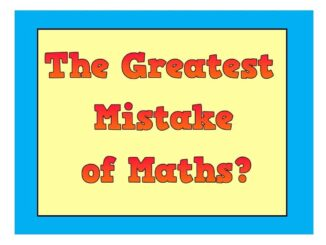THE GREATEST MISTAKE IN THE HISTORY OF MATHS:

This must surely be the exclusion of women from maths for most of our history, due to mathematics (and academia in general) being seen as an unsuitable activity or as simply “too hard” for women. As we see the female:male ratio among the best brains of mathematics now approaching 1:1, one wonders how much moreUSING MATHS TO CHECK WORLD RECORD ATTEMPTS: PETE’S DIABOLO HIGH THROW

My friend Pete can throw a diabolo ridiculously high – check out this video of him in action! Pete thinks his throw is higher than the “official” world record of 23.92metres so asked me if maths could measure his throw. So, how about it, maths? METHOD 1 (not recommended!): get a long tape measure and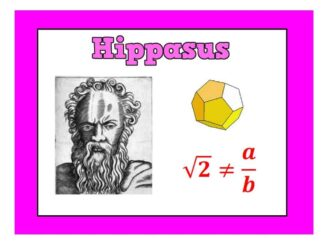HIPPASUS

HIPPASUS: was an Italian born mathematician who lived around 500BC. Legend has it that Hippasus was drowned at sea after daring to suggest that some numbers cannot be written as one whole number divided by another. His pals at the Pythagorean school of maths clearly thought this idea was shocking – but it turns out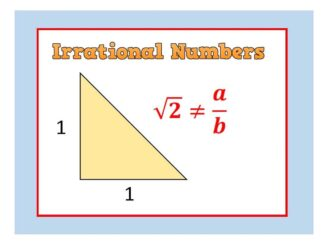Strange but True: IRRATIONAL NUMBERS

The first numbers we discover in childhood are the Natural Numbers: 1, 2, 3, … Next come the Integers (whole numbers) – which also include Zero and the negatives: … -3, -2, -1, 0, 1, 2, 3, … Next up: it’s fractions such as: 0.5, 2.90909… , $-\Large{5}\frac{7}{8}$ Numbers like these are said to beWHY THE ANGLES IN A TRIANGLE ADD TO 180°

Given any triangle: Rotate so that the longest side lies horizontally along the base. Extend the base, and draw a parallel line going through the uppermost corner (these two lines are shown in light blue). The two red angles are the same size (using the well-known “parallel lines” angle result alternate angles, sometimes called “Z-angles“).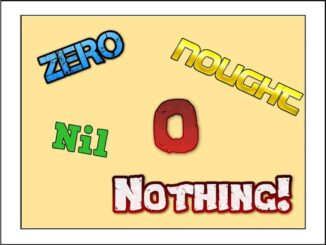(or: Much ado about Nothing) Zero doesn’t actually exist. Think about it: by definition it isn’t anything, it’s nothing!! Even so, here are 5 facts every mathematician should know about Zero: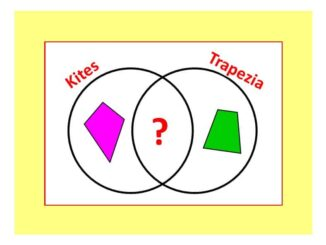PUZZLE OF THE WEEK

What shape goes in the intersection of this Venn Diagram? Read on for the answer: